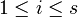# Character determines representation in characteristic zero

## Statement

Suppose$G$ is a finite group and$K$ is a field of characteristic zero. Then, the character of any finite-dimensional representation of$G$ over$K$ completely determines the representation, i.e., no two inequivalent finite-dimensional representations can have the same character.

Note that$K$ does not need to be a splitting field.

## Related facts

### Opposite facts

• Character does not determine representation in any prime characteristic: The problem is that we can construct representations whose character is identically zero simply by adding$p$ copies of an irreducible representation to itself.

## Proof

Given: A group$G$, two linear representations$\rho_1, \rho_2$ of$G$ with the same character$\chi$ over a field$K$ of characteristic zero.

To prove:$\rho_1$ and$\rho_2$ are equivalent as linear representations.

Proof: By Fact (2), both$\rho_1$ and$\rho_2$ are completely reducible, and are expressible as sums of irreducible representations. Suppose$\varphi_1,\varphi_2,\dots,\varphi_s$ is a collection of distinct irreducible representations obtained as the union of all the representations occurring in a decomposition of$\rho_1$ into irreducible representations and a decomposition of$\rho_2$ into irreducible representations. In other words, there are nonnegative integers$a_{11}, a_{12},\dots, a_{1s}, a_{21}, a_{22}, \dots, a_{2s}$ such that:$\rho_1 \cong a_{11}\varphi_1 \oplus a_{12}\varphi_2 \oplus \dots \oplus a_{1s}\varphi_s$

and$\rho_2 \cong a_{21}\varphi_1 \oplus a_{22}\varphi_2 \oplus \dots \oplus a_{2s}\varphi_s$

Let$\chi_i$ denote the character of$\varphi_i$ and denote by$m_i$ the value$\langle \chi_i, \chi_i\rangle_G$ (note: this would be 1 if$K$ were a splitting field, and in general it is the sum of squares of multiplicities of irreducible constituents over a splitting field).

Step no. Assertion/construction Facts used Given data used Previous steps used Explanation
1$m_ia_{1i} = \langle \chi, \chi_i \rangle_G$ and$m_1a_{2i} = \langle \chi, \chi_i \rangle_G$ for each$1 \le i \le s$ Fact (3) Direct application of fact
2$a_{1i} = \frac{1}{m}\langle \chi,\chi_i \rangle_G$ and$a_{2i} = \frac{1}{m} \langle \chi, \chi_i \rangle_G$ for each$1 \le i \le s$$K$ has characteristic zero, so the manipulation makes sense Step (1)
3$a_{1i} = a_{2i}$ for each$1 \le i \le s$$K$ has characteristic zero Step (2) [SHOW MORE]
4$\rho_1$ and$\rho_2$ are equivalent Step (3)